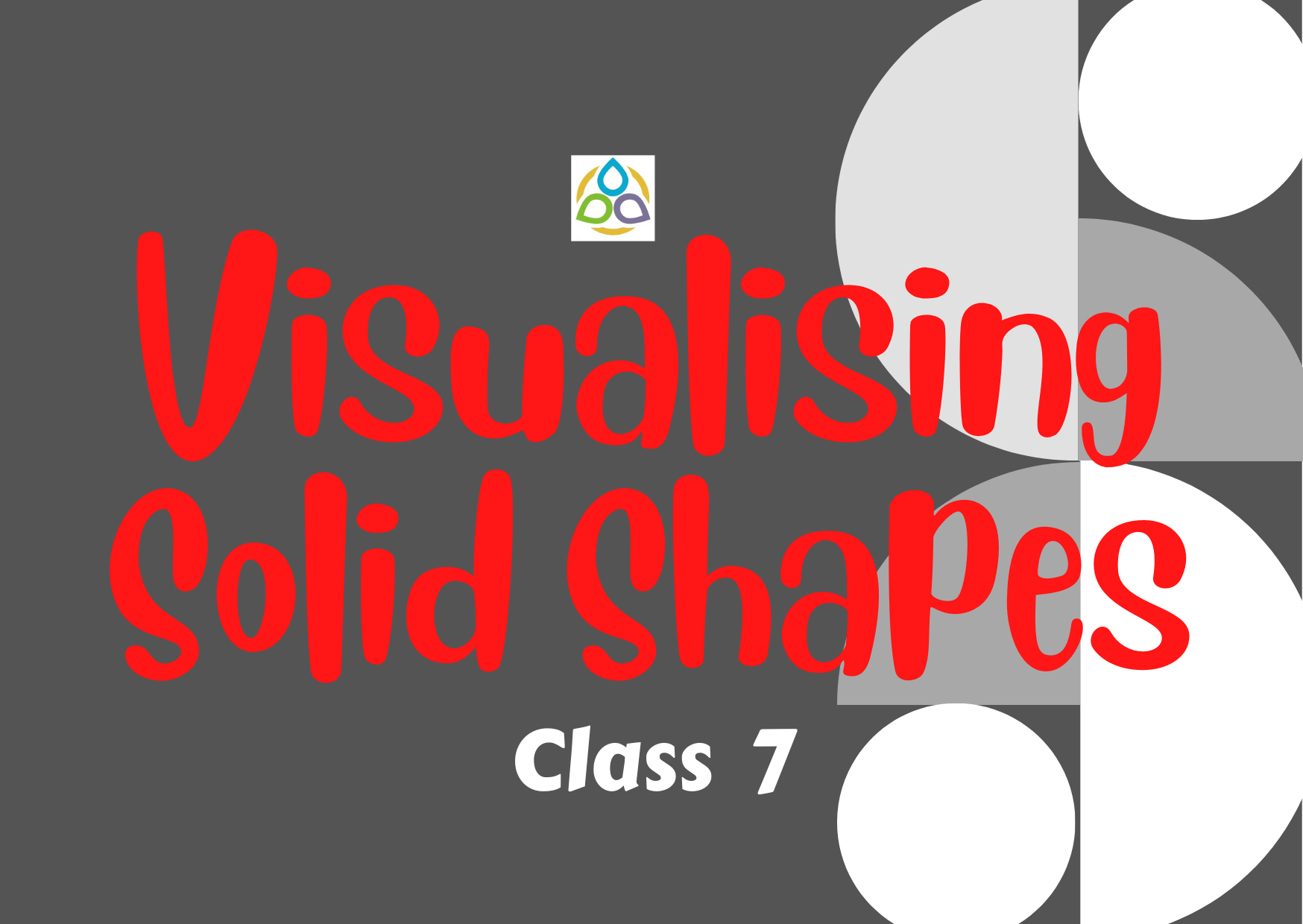# Visualising Solid Shapes – 7

30. What is the value of F + VE in the following figure?

• (A) 1
• (B) 2
• (C) 4
• (D) 3

29. What is the value of F + VE in the following figure?

• (A) 1
• (B) 2
• (C) 4
• (D) 3

28. What is the value of F + VE in the following figure?

• (A) 1
• (B) 2
• (C) 4
• (D) 3

27. Which of the nets given below will generate a cone?

• (A) (i)
• (B) (ii)
• (C) (iii)
• (D) (iv)

26. Which of the following is not a prism?

• (A) (i)
• (B) (ii)
• (C) (iii)
• (D) (iv)

25. Which of the following shapes has a vertex?

• (A) (i)
• (B) (ii)
• (C) (iii)
• (D) (iv)

24. How many faces are there in a pentagonal prism?

• (A) 6
• (B) 7
• (C) 8
• (D) 12

23. What is the number of faces in the following figure?

• (A) 4
• (B) 5
• (C) 6
• (D) 7

22. What is the number of edges in the following figure?

• (A) 8
• (B) 9
• (C) 6
• (D) 7

21. What is the number of vertices in the following figure?

• (A) 4
• (B) 5
• (C) 6
• (D) 7

20. What is the number of faces in the following figure?

• (A) 4
• (B) 5
• (C) 6
• (D) 7

19. What is the number of edges in the following figure?

• (A) 8
• (B) 9
• (C) 6
• (D) 7

18. What is the number of vertices in the following figure?

• (A) 4
• (B) 5
• (C) 6
• (D) 7

17. In the following figure of a cube, which edge is the intersection of faces EFGH and EFBA?

• (A) Triangle
• (B) Square
• (C) Rectangle
• (D) Rhombus

16. In the following figure of a cube, how many faces intersect at the edge EF?

• (A) 0
• (B) 1
• (C) 2
• (D) 3